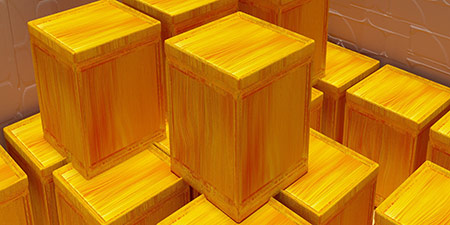# Milliliter (ml) to Liter (l)

Volume ﹣ Converter Milliliter to Liter

Here you can convert the Volume unit Milliliter into the unit Liter and vice versa you can convert Liter into Milliliter. By clicking the "Swap units" icon, you will always obtain the desired conversion in the calculation result, i.e., ml to l or l to ml. With the following calculator you can also calculate any other Volume unit.

One milliliter (ml; spelled millilitre in Commonwealth English and milliliter in American English) corresponds to one cubic centimeter (cm³) and thus to the volume of a cube with an edge length of 1 cm.

The milliliter is not part of the International System of Units (SI), but is approved for use with the SI because it is a fraction of the approved liter.

One liter (l; spelled litre in Commonwealth English and liter in American English) corresponds to one cubic decimeter (dm³) and thus to the volume of a cube with an edge length of 10 cm.

The liter is not part of the International System of Units (SI), but is approved for use with the SI. In addition, the liter is a legal unit of measurement due to national laws.

## Basis for conversion Milliliter (ml) to Liter (l) and vice versa

The abbreviation for the "Volume unit Milliliter" is ml. The abbreviation for the "Volume unit Liter" is l.

## Formula for the conversion of Milliliter (ml) to Liter (l) and vice versa

The calculation from Milliliter to Liter shall be made using the following conversion formula:

Conversion formula Milliliter to Liter

Determine the number of Liter from Milliliter

Milliliter × 0.001

## Formula for the conversion of Liter (l) to Milliliter (ml)

The calculation from Liter to Milliliter shall be made using the following conversion formula:

Conversion formula Liter to Milliliter

Determine the number of Milliliter from Liter

Liter × 1000

## Overview table : How many Milliliter are in a Liter ?

Milliliter ml ⇒ Liter l
0.01 ml  are  0.00001 l
0.02 ml  are  0.00002 l
0.03 ml  are  0.00003 l
0.04 ml  are  0.00004 l
0.05 ml  are  0.00005 l
0.06 ml  are  0.00006 l
0.07 ml  are  0.00007 l
0.08 ml  are  0.00008 l
0.09 ml  are  0.00009 l
0.10 ml  are  0.00010 l
0.20 ml  are  0.00020 l
0.30 ml  are  0.00030 l
0.40 ml  are  0.00040 l
0.50 ml  are  0.00050 l
0.60 ml  are  0.00060 l
0.70 ml  are  0.00070 l
0.80 ml  are  0.00080 l
0.90 ml  are  0.00090 l
1 ml  corresponds to  0.00100 l
2 ml  are  0.00200 l
3 ml  are  0.00300 l
4 ml  are  0.00400 l
5 ml  are  0.00500 l
6 ml  are  0.00600 l
7 ml  are  0.00700 l
8 ml  are  0.00800 l
9 ml  are  0.00900 l
10 ml  are  0.01000 l
20 ml  are  0.02000 l
30 ml  are  0.03000 l
40 ml  are  0.04000 l
50 ml  are  0.05000 l
60 ml  are  0.06000 l
70 ml  are  0.07000 l
80 ml  are  0.08000 l
90 ml  are  0.09000 l
100 ml  are  0.10000 l
200 ml  are  0.20000 l
300 ml  are  0.30000 l
400 ml  are  0.40000 l
500 ml  are  0.50000 l
600 ml  are  0.60000 l
700 ml  are  0.70000 l
800 ml  are  0.80000 l
900 ml  are  0.90000 l
1 000 ml  are  1 l

## Overview table : How many Liter are in a Milliliter ?

Liter l ⇒ Milliliter ml
0.01 l  are  10 ml
0.02 l  are  20 ml
0.03 l  are  30 ml
0.04 l  are  40 ml
0.05 l  are  50 ml
0.06 l  are  60 ml
0.07 l  are  70 ml
0.08 l  are  80 ml
0.09 l  are  90 ml
0.10 l  are  100 ml
0.20 l  are  200 ml
0.30 l  are  300 ml
0.40 l  are  400 ml
0.50 l  are  500 ml
0.60 l  are  600 ml
0.70 l  are  700 ml
0.80 l  are  800 ml
0.90 l  are  900 ml
1 l  corresponds to  1 000 ml
2 l  are  2 000 ml
3 l  are  3 000 ml
4 l  are  4 000 ml
5 l  are  5 000 ml
6 l  are  6 000 ml
7 l  are  7 000 ml
8 l  are  8 000 ml
9 l  are  9 000 ml
10 l  are  10 000 ml
20 l  are  20 000 ml
30 l  are  30 000 ml
40 l  are  40 000 ml
50 l  are  50 000 ml
60 l  are  60 000 ml
70 l  are  70 000 ml
80 l  are  80 000 ml
90 l  are  90 000 ml
100 l  are  100 000 ml
200 l  are  200 000 ml
300 l  are  300 000 ml
400 l  are  400 000 ml
500 l  are  500 000 ml
600 l  are  600 000 ml
700 l  are  700 000 ml
800 l  are  800 000 ml
900 l  are  900 000 ml
1 000 l  are  1 000 000 ml

## Source information

As source for the information in the 'Volume' category, we have used in particular:

## Last update

This page of the 'Volume' category was last edited or reviewed by Stefan Banse on February 20, 2023. It corresponds to the current status.

### Changes in this category "Volume"

• 28.11.2022: Publication of the topic Convert Volumes together with the corresponding texts.
• 28.11.2022: Extension of the Volume Converter by further units: Liquid ounce, cup, tablespoon and teaspoon